Courses

# Test: General Properties of 16th Group (Oxygen, Ozone & Sulphur)

## 25 Questions MCQ Test Chemistry for JEE | Test: General Properties of 16th Group (Oxygen, Ozone & Sulphur)

Description
This mock test of Test: General Properties of 16th Group (Oxygen, Ozone & Sulphur) for JEE helps you for every JEE entrance exam. This contains 25 Multiple Choice Questions for JEE Test: General Properties of 16th Group (Oxygen, Ozone & Sulphur) (mcq) to study with solutions a complete question bank. The solved questions answers in this Test: General Properties of 16th Group (Oxygen, Ozone & Sulphur) quiz give you a good mix of easy questions and tough questions. JEE students definitely take this Test: General Properties of 16th Group (Oxygen, Ozone & Sulphur) exercise for a better result in the exam. You can find other Test: General Properties of 16th Group (Oxygen, Ozone & Sulphur) extra questions, long questions & short questions for JEE on EduRev as well by searching above.
QUESTION: 1

### Direction (Q. Nos. 1-10) This section contains 10 multiple choice questions. Each question has four choices (a), (b), (c) and (d), out of which ONLY ONE is correct. Q.  Which is incorrect statement?

Solution:

In tellurium, differentiating electron enters in to 5p subshell. Its valence shell configuration is 5s25p4.

QUESTION: 2

### In earth’s crust, sulphur exists as

Solution:

Sulphur exists in elemental form which is extracted by Frasch process.
Sulphide ores are zinc blende (ZnS), galena (PbS), copper pyrites (CuFeS2), cinnabar (HgS),
Sulphate ores are gypsum (CaSO4. 2H2O), epsom (MgSO4 .7 H2O) .

QUESTION: 3

### The element used in the production of photocells and solar cells and also used in zerox machines is

Solution:

Se is non-conductor in dark but acts as conductor when exposed to light.

QUESTION: 4

The number of bond pairs and lone pairs in rhombic sulphur molecule are

Solution: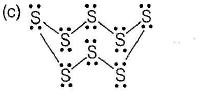QUESTION: 5

Which has lowest bond energy (single bond)?

Solution:

Presence of lone pairs on bonded atoms generally lowers the bond energy.
Bond energy order is
O—O (146) < (S—S) (266) < S—H(366) < O—H(464) kJ/mol

QUESTION: 6

Harmful UV radiations emitted from the sun are prevented from reaching the earth by the presence of ozone in the

Solution:

Stratosphere layer strongly absorb harmful UV-radiations (λ. 255 nm).

QUESTION: 7

Point out the incorrect statement among the following.

Solution:

It is 369 K or 96°C. The stable form at room temperature is rhombic sulphur which transforms to monoclinic sulphur when heated about 369 K.

QUESTION: 8

The correct order of thermal stability of the hydrides of group 16 elements is

Solution: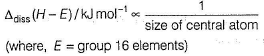Thermal stability ∝ bond dissociation energy
(where, E = group 16 elements)
On moving down the group, bond dissociation energy decreases due to increase in bond length. Thus, the order of bond dissociation energy or thermal stability is
H2O > H2S > H2Se > H2Te > H2PO

QUESTION: 9

When O2 changes to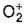Solution: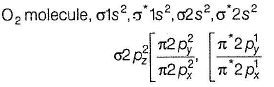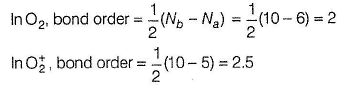QUESTION: 10

Increasing order of oxidation number of oxygen is found in

Solution: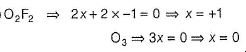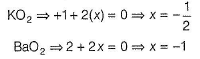*Multiple options can be correct
QUESTION: 11

Direction (Q. Nos. 11-15) This section contains 5 multiple choice questions. Each question has four choices (a), (b), (c) and (d), out of which ONE or MORE THAN ONE are correct.

Q.  Which of the following property do not increase down the group from oxygen to polonium?

Solution:

Electron affinity and ionisation energy decreases down the group due to increase in size.

*Multiple options can be correct
QUESTION: 12

The correct statements among the following are

Solution:

In S8 molecule, sulphur has oxidation state is +2, +4, +6.

*Multiple options can be correct
QUESTION: 13

Which of the following statements regarding ozone are correct?

Solution:

Ozone is thermodynamically unstable w.r.t oxygen since, its decomposition into oxygen results in liberation of heat.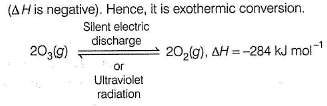*Multiple options can be correct
QUESTION: 14

The correct statements among the following are

Solution:

The correct option is Option A, B and C.

Bond length is inversely proportional to bond order. O2+ has the highest bond order among these three, so it should have the shortest bond length.

Ozone is a powerful oxidizing agent as compared to oxygen. This is due to the unstable nature of ozone and the nascent oxygen that is released during the reaction.

O2 when gas makes others like H2 gas to lose electrons, therefore, O2 gas is an oxidizing agent and H2 when gas loses electrons in redox reaction, therefore H2 gas is a reducing agent.

Oxygen is more stable than ozone. On heating, ozone readily dissociates and forms oxygen and free radicals of oxygen known as nascent oxygen which take part in reaction, thus ozone is more reactive than oxygen

*Multiple options can be correct
QUESTION: 15

Which of the following do not stick to the poles of a magnet?

Solution:

Liquid oxygen is attracted to and actually sticks to the poles of the magnet. It is due to electron distribution as unpaired electrons are present while other do not stick to the poles of magnet.

QUESTION: 16

Direction (Q. Nos. 16 and 17) This section contains a paragraph, each describing theory, experiments, data, etc. Two questions related to the paragraphs have been given. Each question has only one correct answer among the four given options (a), (b), (c) and (d).

Passage

Sulphur and the rest of the elements of 16th group are less electronegative than oxygen. They can acquire ns2np6 by sharing two electrons with the atoms of other elements and thus, exhibit +2 oxidation state in their compounds, in addition to this, atoms have vacant d-orbitals in their valence shell due to which electrons I can be promoted from the s- and p-orbitals of the same shell. As a result, they can show +4 and +6 oxidation states. The oxidation states of elements never exceed +6.

Q.

Oxidation states of sulphur in H2S2O7 and H2S2O8 respectively are

Solution:

H2S2O7 has no peroxy bond while H2S2O8 has one peroxy bond.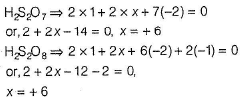QUESTION: 17

Passage

Sulphur and the rest of the elements of 16th group are less electronegative than oxygen. They can acquire ns2np6 by sharing two electrons with the atoms of other elements and thus, exhibit +2 oxidation state in their compounds, in addition to this, atoms have vacant d-orbitals in their valence shell due to which electrons I can be promoted from the s- and p-orbitals of the same shell. As a result, they can show +4 and +6 oxidation states. The oxidation states of elements never exceed +6.

Q.

Like sulphur, oxygen does not show +4 and +6 oxidation states .The reason is

Solution:

Oxygen does not show +4 and +6 oxidation states because oxygen has no cf-orbitals in its valence shell.

QUESTION: 18

Direction (Q. Nos. 18-20) Choices for the correct combination of elements from Column I and Column II are given as options (a), (b), (c) and (d), out of which one is correct.

Q.

Match the Column I with Column II and mark the correct option from the codes given below.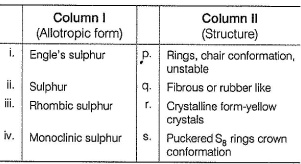Solution: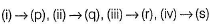QUESTION: 19

Match the Column I with Column II and mark the correct option from the codes given below.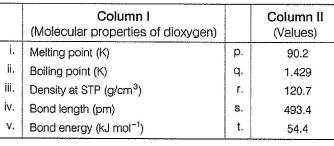Solution: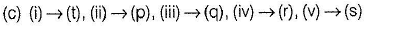QUESTION: 20

Match the Column i with Column II and mark the correct option from the codes given below.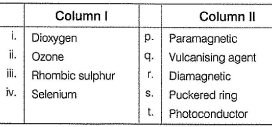Solution: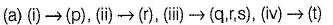*Answer can only contain numeric values
QUESTION: 21

Direction (Q. Nos. 21-24) This section contains 4 questions. When worked out will result in an integer from 0 to 9 (both inclusive).

Q.

Number of chemicals that produce dioxygen on heating

KCIO3, (NH4)2 Cr2O7, NH4NO3, NaNO3, KMnO4, K2Cr2O7, H2O2, Pb3O4, NH4NO2

Solution:

(NH4)2 Cr2O7, NH4NO2 gives dinitrogen while NH4NO3 gives N2O.

*Answer can only contain numeric values
QUESTION: 22

In the fully excited state, number of unpaired electrons in tellurium are

Solution: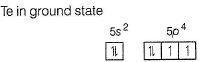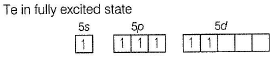*Answer can only contain numeric values
QUESTION: 23

Number of chemical species having bent shape in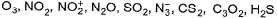Solution:

O3, NO2, SO2 and H2S show bent shape.

*Answer can only contain numeric values
QUESTION: 24

Among the following compounds, the number of compounds which have oxidation states of S is +4 ?

PbS, SO2, SF6, Na2S2O3, H2SO3

Solution:

Let S is in x oxidation state
In PbS, 2 + x = 0 =>  x = -2
In SO2 , x + 2 × (-2) = 0 => x= +4
In SF6 , x + 1 × (-6) = 0 => x = +6
In Na2S2O3, 2 ×(+1) + 2 × x + 3 × (-2) = 0 => x= +2

QUESTION: 25

Direction (Q. No. 25) This section is based on Statement I and Statement II. Select the correct answer from the codes given below.

Q.

Statement I : Oxygen is more electronegative than sulphur, yet H2S is acidic, while H2O is neutral.

Statement II : H— S bond is weaker than O—H bond.

Solution:

H—S bond is weaker than H—O bond hence, H2S is more acidic than H2O.